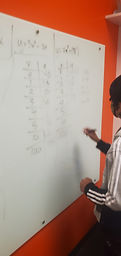## Mr. Jake

### Target 1​

###### Lesson Type:

New

Algebra

:

Function

Use input and output data to determine a function equation.

###### 1:

Define a function as a rule that gives one output to each input.

###### 2:

Study the proportional relationship between inputs and output to derive a function (equation).

###### 3:

Use a function equation to fill out a function table.

###### 4:

Recognize that sequences are functions.

8th

###### Vocabulary:

Activities:

Filled out x/y tables based on a given function

Determined the function that creates a given x/y table### Home Exploration

###### Guiding Questions:## Absent Students:

### Target 2

:

###### 1:

Find the area of triangles, trapezoids, and parallelograms.

###### 2:

Determine the algebraic equations for calculating the area of a triangle (½ base * height), a trapezoid (½ height * (base1 + base2)), and a parallelogram (base * height).

###### 3:

Calculate the area of a shaded region of a figure.

###### 4:

Use previous area understandings (of rectangular figures) to gain insight into the area of new geometric shapes.

8th

###### Vocabulary:

Activities:

Determined the area of trapezoids given the length of both bases and the height### Home Exploration

###### Guiding Questions:### Target 3

:

###### Vocabulary:

Activities:### Home Exploration